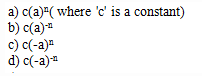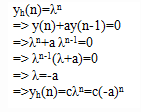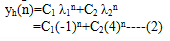# Test: Discrete Time System Implementation

## 10 Questions MCQ Test Digital Signal Processing | Test: Discrete Time System Implementation

Description
Attempt Test: Discrete Time System Implementation | 10 questions in 10 minutes | Mock test for Electrical Engineering (EE) preparation | Free important questions MCQ to study Digital Signal Processing for Electrical Engineering (EE) Exam | Download free PDF with solutions
QUESTION: 1

### If the system is initially relaxed at time n=0 and memory equals to zero, then the response of such state is called as:

Solution:

Explanation: The memory of the system, describes, in some case, the ‘state’ of the system, the output of the system is called as ‘zero-state response’.

QUESTION: 2

### Zero-state response is also known as:

Solution:

Explanation: The zero-state response depends on the nature of the system and the input signal. Since this output is a response forced upon it by the input signal, it is also known as ‘Forced response’.

QUESTION: 3

### Zero-input response is also known as Natural or Free response.

Solution:

Explanation: For a zero-input response, the input is zero and the output of the system is independent of the input of the system. So, the response if such system is also known as Natural or Free response.

QUESTION: 4

The solution obtained by assuming the input x(n) of the system is zero is:

Solution:

Explanation: By making the input x(n)=0 we will get a homogenous difference equation and the solution of that difference equation is known as Homogenous or Complementary solution.

QUESTION: 5

What is the homogenous solution of the system described by the first order difference equation y(n)+ay(n-1)=x(n)?Solution:

Explanation: The assumed solution obtained by assigning x(n)=0 isQUESTION: 6

What is the zero-input response of the system described by the homogenous second order equation y(n)-3y(n-1)-4y(n-2)=0 if the initial conditions are y(-1)=5 and y(-2)=0?

Solution:

Explanation: Given difference equation is y(n)-3y(n-1)-4y(n-2)=0—-(1)
Let y(n)=λn
Substituting y(n) in the given equation
=> λn – 3λn-1 – 4λn-2 = 0
=> λn-22 – 3λ – 4) = 0
the roots of the above equation are λ=-1,4
Therefore, general form of the solution of the homogenous equation isThe zero-input response of the system can be calculated from the homogenous solution by evaluating the constants in the above equation, given the initial conditions y(-1) and y(-2).
From the given equation (1)
y(0)=3y(-1)+4y(-2)
y(1)=3y(0)+4y(-1)
=3[3y(-1)+4y(-2)]+4y(-1)
=13y(-1)+12y(-2)
From the equation (2)
y(0)=C1+C2 and
y(1)=C1(-1)+C2(4)=-C1+4C2
By equating these two set of relations, we have
C1+C2=3y(-1)+4y(-2)=15
-C1+4C2=13y(-1)+12y(-2)=65
On solving the above two equations we get C1=-1 and C2=16
Therefore the zero-input response is Yzi(n) = (-1)n+1 + (4)n+2.

QUESTION: 7

What is the particular solution of the first order difference equation y(n)+ay(n-1)=x(n) where |a|<1, when the input of the system x(n)=u(n)?

Solution:

Explanation: The assumed solution of the difference equation to the forcing equation x(n), called the particular solution of the difference equation is
yp(n)=Kx(n)=Ku(n) (where K is a scale factor)
Substitute the above equation in the given equation
=>Ku(n)+aKu(n-1)=u(n)
To determine K we must evaluate the above equation for any n>=1, so that no term vanishes.
=> K+aK=1
=>K=1/(1+a)
Therefore the particular solution is yp(n)= 1/(1+a) u(n).

QUESTION: 8

What is the particular solution of the difference equation y(n)= 5/6y(n-1)- 1/6y(n-2)+x(n) when the forcing function x(n)=2n, n≥0 and zero elsewhere?

Solution:

Explanation: The assumed solution of the difference equation to the forcing equation x(n), called the particular solution of the difference equation is
yp(n)=Kx(n)=K2nu(n) (where K is a scale factor)
Upon substituting yp(n) into the difference equation, we obtain
K2nu(n)=5/6K2n-1u(n-1)-1/6 K2n-2u(n-2)+2nu(n)
To determine K we must evaluate the above equation for any n>=2, so that no term vanishes.
=> 4K= 5/6(2K)-1/6 (K)+4
=> K= 8/5
=> yp(n)= (8/5) 2n.

QUESTION: 9

The total solution of the difference equation is given as:

Solution:

Explanation: The linearity property of the linear constant coefficient difference equation allows us to add the homogenous and particular solution in order to obtain the total solution.

QUESTION: 10

What is the impulse response of the system described by the second order difference equation y(n)-3y(n-1)-4y(n-2)=x(n)+2x(n-1)?

Solution:

Explanation: The homogenous solution of the given equation is yh(n)=C1(-1)n+C2(4)n—-(1)
To find the impulse response, x(n)=δ(n)
now, for n=0 and n=1 we get
y(0)=1 and
y(1)=3+2=5
From equation (1) we get
y(0)=C1+C2 and
y(1)=-C1+4C2
On solving the above two set of equations we get
C1=- 1/5 and C2= 6/5
=>h(n)= [-1/5 (-1)n + 6/5 (4)n]u(n).Use Code STAYHOME200 and get INR 200 additional OFF Use Coupon Code